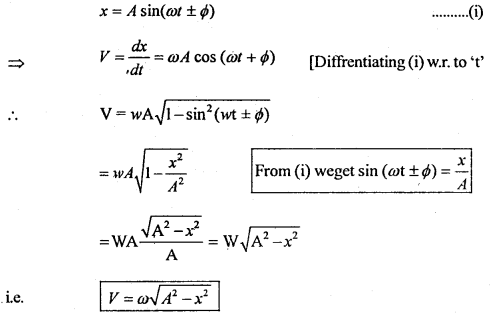Here we are providing Class 11 Physics Important Extra Questions and Answers Chapter 14 Oscillations. Important Questions for Class 11 Physics with Answers are the best resource for students which helps in Class 11 board exams.

## Class 11 Physics Chapter 14 Important Extra Questions Oscillations

### Oscillations Important Extra Questions Very Short Answer Type

Question 1.
(a) A particle has maximum velocity in the mean position and zero velocity at the extreme position. Is it a sure test for S.H.M.?
No.

(b) Is restoring force necessary in S.H.M.?
Yes.

Question 2.
Imagine a situation where the motion is not simple harmonic but the particle has maximum velocity in the mean position and zero velocity at the extreme position.
Projection of a particle in non-uniform circular motion satisfies all the given conditions.

Question 3.
We know that in S.H.M., the time period is given by
T = 2π$$\sqrt{\frac{\text { Displacement }}{\text { Acceleration }}}$$
Does T depend upon displacement?
No. T is independent of displacement as the displacement term is also contained in acceleration.

Question 4.
(a) Two simple pendulums of equal lengths cross each other at the mean position. What is their phase difference?

(b) Can a simple pendulum vibrate at the center of Earth? Why?
No. This is because the value of g at the center of Earth is zero.

Question 5.
(a) A particle is in S.H.M. of amplitude 2 cm. At the extreme position, the force is 4N. What is the force at a mid-point i.e. midway between mean and extreme position?
2N.

(b) What happens to the time period of a simple pendulum if its length is doubled?
The time period is increased by a factor of 4l.

Question 6.
Can & simple pendulum be used in an artificial satellite? Why?
No. This is because there exists a state of weightlessness in the artificial satellite.

Question 7.
What fraction of the total energy is potential energy when the displacement is one-half of the amplitude?
$$\frac{1}{4}\left[\because \frac{P . E}{\text { Total energy }}=\frac{\frac{1}{2} \operatorname{m\omega}^{2}\left(\frac{a}{2}\right)^{2}}{\frac{1}{2} m \omega^{2} a^{2}}\right]$$

Question 8.
What fraction of the total energy is kinetic energy, when the displacement is one-half of the amplitude?
$$\frac{3}{4}\left[\because \frac{\text { K.E. }}{\text { Total energy }}=\frac{\frac{1}{2} m \omega^{2}\left(a^{2}-\left(\frac{a}{2}\right)^{2}\right)}{\frac{1}{2} m \omega^{2} a^{2}}=\frac{3}{4}\right]$$

Question 9.
(a) When a particle oscillates simply harmonically, its potential energy varies periodically. If v be the frequency of oscillation of the particle, then what is the frequency of vibration of P.E.?
2v

(b) A body executes S.H.M. with a period of $$\frac{11}{7}$$ seconds and an amplitude of 0.025 m. What is the maximum value of acceleration?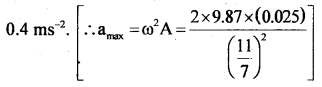Question 10.
A body of mass m when hung on a spiral spring stretches it by 20 cm. What is its period of oscillation when pulled down, and released?
T = 2π$$\sqrt{\frac{20}{980}}=\frac{2 \pi}{7} \mathrm{~s}=\frac{44}{49} \mathrm{~s}$$

Question 11.
A spring-mass system oscillating vertically has a time period T. What shall be the time period if oscillating horizontally?
T i.e. it remains the same.

Question 12.
The time period of a body executing S.H.M. is 0.05 s and the amplitude of vibration is 4 cm. What is the maximum velocity of the body?
1.6 π ms-1

Question 13.
A spring-controlled wristwatch is taken from Earth to Moon. What shall be the effect on the watch?
There will be no effect on g as the time period of spring is independent of g.

Question 14.
(a) At what displacement, the P.E. of a simple harmonic oscillator is maximum?
At the extreme position.

(b) At what displacement, the K.E. of a simple harmonic oscillator is maximum? ‘
At the mean position.

Question 15.
What is the total energy of a simple harmonic oscillator?
$$\frac{1}{2}$$m ω2 r2, where r = amplitude, ω = angular frequency, m = mass of the oscillator.

Question 16.
Name the trigonometric functions which are suitable for the analytical treatment of periodic motions. ,
Sine and Cosine functions or their linear combination.

Question 17.
How is acceleration (a) related to the displacement (y) in S.H.M.?
aα – y.

Question 18.
At what position, the velocity of a particle executing S.H.M. is maximum?
At mean position.

Question 19.
What is Force constant (k)? What are its units in the S.I. system?
k = Force per unit displacement.
S.I unit of k is Nm-1

Question 20.
(a) What ¡s the phase difference between displacement and velocity of a particle executing S.H.M.?
90° or $$\frac{π}{2}$$ radian.

(b) What is the phase difference between displacement and acceleration of a particle executing S.H.M.?

Question 21.
(a) What will be the change in the time period of a loaded spring when taken to Moon?
No change because T = 2π$$\sqrt{\frac{m}{k}}$$ i.e. T ¡s independent of g.

(b) Why does the time period (T) of the swing not change when two boys sit on it instead of one?
T is independent of the mass of the swing as T = 2π$$\sqrt{\frac{l}{g}}$$.

Question 22.
Why are the vibrations of a simple pendulum damped?
Because the energy of the pendulum is used to overcome air resistance.

Question 23.
A pendulum clock is taken on a lift moving down with a uniform Velocity. Will it gain dr lose time?
It neither gains nor loses time.

Question 24.
What role do shock absorbers play in oscillations of vehicles on a bumpy road?
They act as damping devices and save vehicles from resonant oscillations.

Question 25.
When do you apply force as the spring descends while swinging on a swing?
To counter the effect of the damping force on the amplitude of oscillations of the swing at an appropriate point.

Question 26.
The time period of oscillation of a sphere hung from a wire attached to a rigid support and given slight rotation about the wire is given by T = 2π$$\sqrt{\frac{I}{C}}$$. Which factor out of these is equivalent to the mass factor of an oscillator in S.H.M.?
I (moment of inertia) of the sphere is equivalent to m, the mass of an ordinary oscillator in S.H.M.

Question 27.
At what points is the energy entirely K.E. and entirely P.E. in S.H.M.?
At mean position and extreme position respectively.

Question 28.
What provides the restoring force for simple harmonic motion in the following cases?
(i) Simple pendulum.
(ii) Spring.
(iii) Column of mercury in a U-tube.
The restoring force is provided by:
(a) gravity in simple pendulum i.e. weight of the pendulum.
(b) elasticity in spring.
(c) weight in the column of Hg in a U-tube.

Question 29.
Which single characteristics of a periodic motion distinguishes it as S.H.M?
If acceleration is directly proportional to the negative- displacement, then the motion is said to be S.H.M.

Question 30.
(a) Does the bursting of air bubbles at the surface of boiling water show a periodic motion of the surface molecules?
No.

(b) Do all periodic motions are S.H.M.?
No.

Question 31.
At what point the velocity and acceleration are zero in S.H.M.?
The velocity is zero at the extreme points of motion and acceleration is zero at the mean position of motion.

Question 32.
On what factors does the force constant of a spring depend?
It Depends Upon the following factors:

1. Nature of the material of the spring.
2. Elasticity (i.e. coefficient of elasticity) of the material of spring.

Question 33.
Which factors determine the natural frequency of an oscillator?
The dimensions and the elastic properties of the oscillator.

Question 34.
When a high-speed plane passes by, the windows of the plane start producing sound due to
(i) S.H.M.,
(ii) damped oscillations,
(iii) maintained oscillations. Why?
(ii) Damped oscillations are caused due to air pressure on the glass window panes.

Question 35.
A motorcyclist while trying to loop a loop of maximum radius in a death trap goes round in the globe. Is it his projection on diameter in S.H.M.?
Yes.

Question 36.
Sometimes on the screen of a cathode-ray oscilloscope, we see rectangular or triangular waves. Are these formed due to S.H.M. of the oscillator connected to it?
No, these are not S.H.M. but are periodic waves.

Question 37.
Is oscillation of a mass suspended by a spring simple harmonic?
Yes, only if the spring is perfectly elastic.

Question 38.
A diver wearing an electronic digital watch goes down into seawater with terminal velocity v. How will the time in the waterproof watch be affected?
It will not be affected as its action is independent of gravity and buoyant force.

Question 39.
When is the tension maximum in the string of a simple pendulum?
The tension in the string is maximum at the mean position because the tension in the string = mg cos θ = mg. (∵ θ = 0 at mean position).

Question 40.
Is the damping force constant on a system executing S.H.M.?
No, because damping force depends upon velocity and it is more when the system moves fast and is less when the system moves slow.

Question 41.
What is the (a) distance covered by (b) displacement of, a body executing S.H.M. in a time equal to its period if its
amplitude is r?
A body executing S.H.M. completes one vibration in a time equal to its period, so the body reaches its initial position after a time equal to its period.
Thus the
(a) total distance traveled is 4r and
(b) displacement is zero.

Question 42.
Can a motion be oscillatory but not simple harmonic? If yes give an example and if no explain, why?
Yes. Uniform circular motion is an example of it.

Question 43.
How will the period of a pendulum change when its length is doubled?
The time period becomes $$\sqrt{2}$$ times the original value as T ∝ $$\sqrt{l}$$.

Question 44.
Determine whether or not the following quantities can be in the same direction for a simple harmonic motion;
(a) displacement and velocity,
Yes, when the particle in S.H.M. is moving from equilibrium position to extreme position.

(b) velocity and acceleration,
Yes, when the particle executing S.H.M. is moving from an extreme position to a mean position.

(c) acceleration and displacement?
No, because acceleration is always directed opposite to the displacement.,

Question 45.
Can a body have zero velocity and maximum acceleration in S.H.M.?
Yes. In S.H.M., at the extreme position, the velocity of the body is zero and acceleration is maximum.

Question 46.
A passing airplane sometimes causes the rattling of windows of a house. Explain why?
When the frequency of the sound waves produced by the engine of the airplane strikes the windows panes, they start vibrating due to forced oscillations. This causes rattling of windows.

Question 47.
Identify periodic, non-periodic, and S.H.M. out of the following:
(a) fluttering tree leaves due to wind.
Non-periodic

(b) fluttering of honeybee’s wings for a microsecond.
S.H.M.

(c) rising and falling of water drops at the bottom of a waterfall.
Non-periodic

(d) the sound produced by a motorcycle in a small interval of time.
Periodic

(e) the motion of the wheels of a canon during rapid fire.
Periodic

(f) jerks in a machine gun during rapid fire.
periodic

(g) vibrations of a drum membrane in a music band.
Non-periodic.

Question 48.
Why pendulum clocks are not suitable for spaceships?
The time period of a pendulum clock is given by
T = 2π$$\sqrt{\frac{l}{g}}$$

In a spaceship g = 0, so T = ∞,
It means the pendulum clock will not oscillate and hence are not suitable for spaceships.

Question 49.
A glass window may be broken by a distant explosion. Is it correct?
Yes. The sound waves can cause forced vibrations in glass due to the difference between the frequency of sound waves and the natural frequency of the glass. This can break the glass window.

Question 50.
The bob of a simple pendulum is positively charged. The pendulum is made to oscillate over a negatively charged plate. How shall the time period change as compared to the natural time period of the simple pendulum?
Due to the electric force of attraction between the bob and the plate, the effective value of acceleration due to gravity will increase T = 2π$$\sqrt{\frac{l}{g}}$$ therefore T will decrease.

### Oscillations Important Extra Questions Short Answer Type

Question 1.
Why does the body of a bus begin to rattle sometimes when the bus is accelerated?
At some speed of the bus, the frequency of vibrations produced by the bus-engine is equal to the natural frequency of the bus. This produces resonant vibrations which have quite a large amplitude and\ence the bus begins to rattle.

Question 2.
The displacement of a particle in S.H.M. may be given by x = A sin (ωt + Φ) Show that if the time t is increased by $$\frac{2π}{ω}$$, the value of x remains the same.
The displacement of a particle in S.H.M. at a time t is given
x = A sin (ωt + Φ) …. (i)

Let x be the displacement of the-particle at time t’ = ( t + $$\frac{2π}{ω}$$),
To prove x1 = x

From equation (i), we get
x1 = A sin [ω( t + $$\frac{2π}{ω}$$) + Φ)]
= A sin [ωt + 2π + Φ]
= A sin [2π + (ωt + Φ)]
= A sin (ωt + Φ)
[ ∵ sin (2π + θ) = sin θ]

i.e. the displacement of the particle in S.H.M. is same for times t and t + $$\frac{2π}{ω}$$ .
Hence proved.

Question 3.
A hollow sphere is filled with water through a small hole in it. It is hung by a long thread and as water slowly flows out of the hole at the bottom, one finds that the period of oscillations first increases and then decreases. Explain why?
When the sphere is filled with water, its C.G. is at the center of the sphere. We know that the time period (T) of oscillation is directly proportional to the square root of the effective length of the pendulum
i.e. T ∝ $$\sqrt{l}$$.

Due to the flow out of the water through the hole at the bottom of the sphere, the first effective length l increases, and then it decreases because of the lowering of the center of gravity in the first case and rising up in the second case. The first case corresponds to the lowering of the water level up to the center of the sphere. The second case corresponds to the lowering of the water level from the center of the sphere to its bottom.

Question 4.
A girl is swinging in the sitting position. How will the period ^ of the swing be changed if she stands up?
This can be explained using the concept of a simple pendulum.
We know that the time period of a simple pendulum is given by
T = 2π $$\sqrt{\frac{l}{g}}$$ i.e. T ∝ $$\sqrt{l}$$

When the girl stands up, the distance between the point of suspension arid the center of mass of the swinging body decreases i. e. l decreases, so l will also decrease.

Question 5.
At what displacement, a particle in S.H.M. possesses half K.E. and half P.E.?
We know that in any position for a displacement of y from the mean position, the K.E. and P.E. are given by the expressions:
Ek = $$\frac{1}{2}$$ mω2 (r2 – y2) ….(1)
Ep = $$\frac{1}{2}$$mω2y2 …..(2)
Where m = mass of the particle
y = displacement from the mean position
ω = its angular frequency
r = amplitude of oscillation of the particle

Now for Ek = Ep, we get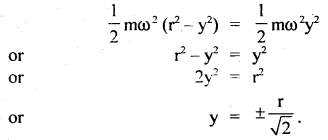Question 6.
Explain why marching troops are asked to break their steps while crossing a bridge?
This is done so as to avoid setting the bridge into resonant oscillations of large amplitude. If troops march in step, then the frequency of the steps of the marching troops may become equal to the natural frequency of vibration of the bridge. Hence it may oscillate with high amplitude due to the phenomenon of resonance, resulting in damage to the bridge.

Question 7.
What is the direction of acceleration at the mean and extreme positions of an oscillating simple pendulum?
When the simple pendulum oscillates, its bob moves along a circular path with the point of suspension at its center. At the mean position, bob possesses centripetal acceleration which acts along the thread towards the point of suspension. At an extreme position, the bob is at rest for a moment. The acceleration on the bob acts along the tangent to the circular path and is directed towards its mean position. This acceleration results due to the restoring force arising due to the weight of the bob.

Question 8.
You are provided with a light spring, a meter scale, and a known mass. How will you find the time period of oscillation of mass attached to the spring without the use of a clock?
With the help of metre scale, we can note the length and extension produced. Since
F = – kl
and F = mg
or
∴ mg = – k l
or
$$\frac{\mathrm{m}}{\mathrm{k}}=\frac{l}{\mathrm{~g}}$$ (numerically)
∴ T = 2π$$\sqrt{\frac{m}{k}}$$ = 2π$$\sqrt{\frac{l}{g}}$$ …(1)

∴ By knowing the extension l, T can be calculated from equation (1) w ithout the use of clock.

Question 9.
Why does the time period of a pendulum change when taken to the top of a mountain or deep in a mine? Will clocks keep the correct time?
Time period of a pendulum is given by
T = 2π$$\sqrt{\frac{l}{g}}$$
As the value of ‘g’ on the top of a mountain or deep in a mine decreases, so the time period of the pendulum increases on the top of a mountain or deep in a mine and hence the pendulum clocks will run slow,

Question 10.
What is the source of potential energy in a loaded elastic spring?
As the load is put at the end of the spring, it gets extended. To increase the length, the load does work against the elastic restoring force. This work done is stored in the spring in the form of its potential energy,

Question 11.
Why cannot we construct an ideal simple pendulum?
An ideal simple pendulum is made up of a heavy point mass suspended to a weightless, inextensible string fastened to a rigid support. In actual practice geometrical point mass is not realized so is a weightless and inextensible string. Hence ideal simple pendulum cannot be constructed in actual practice.

Question 12.
When a fast railway train passes over a river bridge, we hear a loud sound, why?
Fast railway train vibrations are passed to the air column between the bridge and the river water through railway lines and slippers. The vertical air column starts resonating with train vibrations, so a loud sound is heard. ‘

Question 13.
If the amplitude of vibration of an oscillator is made half, how will its time period and energy be affected?
Since the time period of an oscillator is independent of its amplitude, so it remains unaffected. However, the total energy is directly proportional to the square of the amplitude, so the energy of the oscillator will become a quarter of its original value on making amplitude half.

Question 14.
A bead is mounted on a vertical stand placed attached to the rim of a record player and its shadow is cast on the wall with the help of light from a lamp. The record player is set in motion. Describe the motion of the shadow of the bead.
As the bead executes circular motion with the record player, its shadow on the wall will execute whose period depends on the speed of rotation of the record player.

Question 15.
In Boyle’s law apparatus, if the open end tube is quickly moved up and down what will happen to the mercury column in the closed tube?
Due to quick compression and expansion of air column above mercury column in the closed tube, the mercury column is set into damped harmonic motion. Damping is caused due to friction in the air layer and mercury with the glass wall.

Question 16.
Alcohol in a U-tube is executing S.H.M. of time period T. Now alcohol is replaced by water up to the same height in U-tube. What will be the effect on the time period?
As the time period (T) of oscillation of a liquid column in a U-tube does not depend upon the density of the liquid, so T is independent of the nature of liquid in a U-tube, hence there will be no effect on the time-period of replacing alcohol by water.

Question 17.
A spring having a force constant k and a mass m is suspended. The spring is cut into three halves and the \$ame mass is suspended from one of the halves. Is the frequency of vibration the same before and after the spring is cut? Give reason.
No. This can be explained as follows:
The spring constant k = $$\frac{F}{x}$$, when the spring is cut inito three parts, the hew force constant will be k’ = $$\frac{\mathrm{F}}{\frac{\mathrm{x}}{3}}=\frac{3 \mathrm{~F}}{\mathrm{x}}$$ = 3k.

If v = frequency of oscillation when the spring was not divided,
Then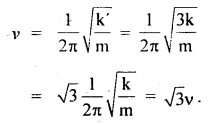i.e. frequency of oscillation after cutting the spring into 3 parts is $$\sqrt{3}$$ times the frequency of oscillation before cutting. It is now clear to us that this is due to the reason that v ∝ $$\sqrt{k}$$ and k increases on cutting the spring, so frequency increases.

Question 18.
Oscillatory motion is periodic but the reverse is not always true. Justify.
The motion of planets and comets like Hailey’s comet, satellites, merry-go-round, etc. all are periodic but not oscillatory as these are not to and fro motions about a mean position. But the motion of the simple pendulum is not only oscillatory but periodic too as it is also to and fro and repeats itself at regular intervals of time.

Question 19.
Why a restoring force is a must for S.H.M.?
A restoring force is a force that restores or tends to restore or bring back a body or particle to its equilibrium position. When a body executing S.H.M. crosses its mean position due to its kinetic energy, the restoring force starts acting towards the equilibrium position and brings the oscillator towards the mean position from the extreme position of motion. The same thing happens when the body goes to the other side of the equilibrium position. So, a restoring force is a must for any oscillatory motion including S.H.M.

Question 20.
What is a periodic function, explain?
A mathematical function that repeats itself after a definite period is called a periodic function, e.g. f (t) = f (t + T), here T = period of the function.

Let F(t) fie a periodic function such that F(t) = A sin $$\frac{2π}{T}$$ t or F(t) = A cos $$\frac{2π}{T}$$ t which are periodic functions. If t is replaced by t + T, we get once again the same value of the function. Thus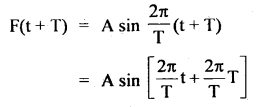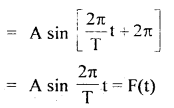Hence f(t) is a periodic function having a time period T.

Question 21.
Distinguish between forced vibrations and maintained vibrations.
A body vibrating under the effect of an external applied periodic force and oscillating with a frequency other than its natural frequency is said to be performing forced vibrations.

If energy is supplied to the oscillator at the same rate at which it is dissipated, the amplitude of the oscillator remains unchanged. Such oscillations are called maintained oscillations e.g. The oscillations of the pendulum clock of a watch or the balance wheel of a watch are examples of maintained oscillations.

Question 22.
Will a pendulum go slower or faster at
(a) Moon,
(b) planet Jupiter?
The time period of oscillation of a pendulum on Earth is given by
Te = 2π$$\sqrt{\frac{1}{g_{e}}}$$
(a) On moon gm = $$\frac{1}{6}$$ of the Earth = $$\frac{\mathrm{g}_{\mathrm{c}}}{6}$$.Since gm < ge, so Tm > Te. Thus due to increase in time period of oscillation of pendulum, it will go slower on the moon.

(b) The value of acceleration due to gravity gj on Jupiter is more than ge, so the time period TJ of oscillation of the pendulum will be less than what it is on the earth. Thus due to a decrease in the time period of oscillation of a pendulum, it will go faster on Jupiter.

Question 23.
The mass M attached to a spring oscillates with a period of 2s. If the mass is increased by 2 kg, the period increases by 1 s. Find the initial mass m assuming that Hooke’s law is obeyed.
Let the initial mass and time period be M and T respectively. If the Hook’s law is obeyed, then the oscillations of the spring will be simple harmonic having time period T given by
T = 2π$$\sqrt{\frac{M}{k}}$$

Given T = 2s
∴ 2 = 2π$$\sqrt{\frac{M}{k}}$$; k = spring constant ….(1)

On increasing the mass by 2 kg, T’ = 2 + 1 = 3s.
∴ 3 = 2π$$\sqrt{\frac{M+2}{k}}$$

Squaring and dividing equation (2) by (1), we have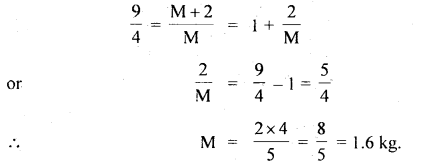Question 24.
The displacement of a particle having periodic motion is given by
y = r sin ωt
Show that the motion of the particle is simple harmonic for small values of v.
Since y = r sin(ωt – Φ) ….(1)
∴ $$\frac{dy}{dt}$$ = rω cos(ωt – Φ) …(2)
and $$\frac{\mathrm{d}^{2} \mathrm{y}}{\mathrm{dt}^{2}}$$ = – rω2 sin(ωt – Φ)
= – ω2 y …(3) [by (i)]

where,a = $$\frac{\mathrm{d}^{2} \mathrm{y}}{\mathrm{dt}^{2}}$$ is called acceleration.
ω = angular frequency
∴ a = – ω2y

Now as a ∝ y and acts towards mean position. This is the characteristic of S.H.M. Hence the given equation y = r sin (ωt – Φ) represents an S.H.M. of the oscillator.

Question 25.
We have a very strong string and of sufficient length making a pendulum with a metal ball (e.g. a shot put). What will be the effect on the time period if
(a) the length of the pendulum is doubled and
If the length of the pendulum is doubled, then
T = 2π$$\sqrt{\frac{2l}{g}}$$
= 2π$$\sqrt{\frac{l}{g}}$$ $$\sqrt{2}$$ = $$\sqrt{2}$$T.
The period of oscillation will increase.

(b) the ball is replaced by an elephant?
Since mass does not come in relation to the time period of oscillation of a pendulum therefore instead of shot put if we make a pendulum of some length with an elephant there will be no effect on the time period of its oscillation.

Question 26.
The angular frequency of damped harmonic motion is given by ω = $$\sqrt{\frac{k}{m}-\left(\frac{b}{2 m}\right)^{2}}$$ where b is know as damping constant. The displacement in such a motion is given by x = A e-bt/2m cos (ωt + Φ) and the retarding force is F = – b v, where v is the speed of the particle. Could you guess from the given equations?
(a) How the amplitude of vibration change?
The amplitude of vibration decreases continuously due to damping force. The amplitude decreases exponentially as is evident from the displacement equation the damping force is velocity-dependent.

(b) Does the time period of vibration change with displacement?
The time period of oscillation does not change in damped oscillations.

Question 27.
Apart from the danger of being burnt which other factors make the re-entry of a space vehicle difficult in Earth’s atmosphere?
The Earth’s thick atmospheric layer offers buoyant force and space vehicles suffer from damped harmonic oscillations, making the re-entry difficult.

Question 28.
If the amplitude of vibration is not small in the simple pendulum experiment will it not be executing periodic harmonic oscillations? How will the time period be affected?
The simple pendulum will still be executing periodic harmonic oscillation even if the amplitude is large. However, the time period of oscillation will be slightly greater than what it is for small amplitudes
i.e. T > 2π$$\sqrt{\frac{l}{g}}$$ ,

(The derivation of formulae is beyond the scope of this course),

Question 29.
Can you give some examples where an anharmonic oscillator does not possess potential energy?
The thermal radiation enclosed in a black body of fixed dimensions and photons in solids behave like harmonic oscillators where they don’t have potential energy.

Question 30.
A man is standing on a platform executing S.H.M. in the vertical direction and attached to a weighing machine. Will there be any change in his weight?
Depending on the motion of the platform from its mean position, the weight of the man will change when the platform moves down from the mean position to the lowermost point and return back to the mean position, ‘ the acceleration acts vertically upwards and hence the weight of the man j will increase. But when the platform moves up from its mean position to [ the uppermost point and returns back to the mean position, the acceleration in S.H.M. acts downwards, so the weight of the man is decreased.

### Oscillations Important Extra Questions Long Answer Type

Question 1.
(a) What is the effect on the time period of a simple pendulum when:
(i) it is immersed in a liquid of density σ.
Let p = density of the material of the pendulum bob.
σ = density of the liquid in which the pendulum bob is immersed such that p > σ. Let it is made oscillate with a time period T’. The effective value of ‘g’ in the liquid is given bywhere T = time period of the simple pendulum when it oscillates in air.
or
$$\frac{\mathrm{T}^{\prime}}{\mathrm{T}}=\sqrt{\frac{\rho}{\rho-\sigma}}$$
∴ ρ > σ
or
ρ – σ > 0

∴ $$\frac{\rho}{\rho-\sigma}$$ > 1
or
$$\frac{\mathrm{T}^{\prime}}{\mathrm{T}}$$ > 1
or
T’ > T
i.e. its time period increases.

(ii) the temperature of wire through which the bob is suspended increased?
When the temperature of the wire with which the bob of the pendulum is suspended increases, the length of the wire increases due to its thermal expansion. As
T ∝ $$\sqrt{l}$$, so T will also increase as shown below:
Let l = original length of the wire
dθ = rise in its temperature
α = coefficient of linear expansion of the material of the wire
If l’ = new length
and dl = increase in its length = l’ – l, then(Using Binomial expansion)
or increase in Time period = T’ – T = $$\frac{\mathrm{T} \alpha \mathrm{d} \theta}{2}$$

(b) Derive the differential equation of Simple Harmonic Oscillation.
When an oscillator is displaced, say in the x-direction, the restoring force tends to bring it in its equilibrium position. It is well known that restoring force is directly proportional to the displacement, thus
Fα – x or F = – k x(t) .
where, the negative sign indicates! that the force always acts towards equilibrium position and opposes displacement, k is known as the force constant.

According to Newton’s law of motion if x (t) is the displacement then $$\frac{\mathrm{dx}(\mathrm{t})}{\mathrm{dt}}$$ is the velocity and $$\frac{\mathrm{d}^{2} \mathrm{x}(\mathrm{t})}{\mathrm{dt}^{2}}$$ is the acceleration of the oscillating particle.

Now Force = mass × acceleration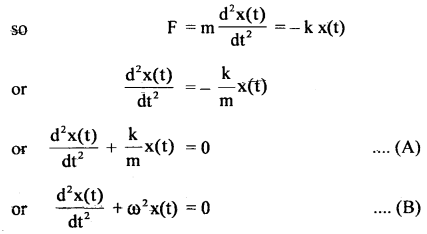Equations (A) and (B) are known as the differential equations of simple harmonic oscillator, GO is the angular velocity given by
ω = $$\frac{2π}{T}$$
Also
ω = $$\sqrt{\frac{k}{m}}$$

So, the time period of oscillation of the oscillator is given by
$$\frac{2π}{T}$$ = $$\sqrt{\frac{k}{m}}$$
or
T = 2π$$\sqrt{\frac{m}{k}}$$

(c) Distinguish briefly between natural, forced and , resonant vibrations. What are damped and undamped / vibrations?
Natural vibrations: The vibrations of a body in the absence of any resistive, damping, or external) forces are said to be its natural vibrations. The body once vibrated continues to vibrate with its natùral frequency and undiminished amplitude.

Forced vibrations: A body at rest or vibrating with some frequency changes its frequency of vibration and amplitude when an external force is impressed on it. If the applied force is periodic the body oscillates with a period which is the period of the impressed force.

The vibrations of the body are not natural or free vibrations but are forced vibrations. If the external periodic force is removed, the body executes its natural oscillations after a while.

Resonant vibrations: If a body is set in vibrations such that a periodic force creates vibrations in it and the frequency of such vibrations becomes equal to the natural frequency of vibration of the body (i.e. the frequency of its free vibrations in the absence of the applied periodic force), its amplitude of vibration increases vigorously. The body is said to be in resonance with the second oscillating body or the applied periodic force.

The frequencies of the two oscillators become equal. Resonant oscillations are not only set up in mechanical oscillators but in electrical circuits and atomic oscillators too.

Damped vibrations: If a body vibrates in the presence of a resistive force such as fluid friction or electromagnetic damping force, its amplitude of oscillation decreases, and ultimately the oscillations die out i.e. the body stops oscillating. Such an oscillation is called damped oscillation as shown in the figure. The amplitude of vibration decreases with time.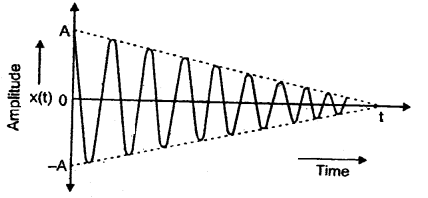Undamped Oscillations: The free oscillations of an oscillator in the absence of any resistive or damping force have constant amplitude over time. Such oscillations are called undamped oscillations. Most vibrations in ‘ daily life are damped vibrations because the oscillator experiences one or the other type of force acting on it. So, its vibrations become damped.

Question 2.
(a) Discuss the effect of driving force frequency on the driven frequency. How does friction affect the oscillation of an oscillator?
Driving force is a time-dependent force represented by
F(t) = fo cos 2πvt …..(1)
where v = frequency of driving force. This frequency is different from the natural frequency of the oscillation vn of the oscillator. vn is also called driven frequency. Thus motion depends on driving force.

Thus the motion of the particle is now under the action of: (a) linear restoring force, (b) time dependent (or periodic) force given in equation (1) so that using Newton’s law, we have for the oscillator:
ma(t) = – k x(t) + fo cos2πvt …. (2)
and vn2 = $$\frac{1}{\mathrm{~T}^{2}}=\frac{\mathrm{k}}{4 \pi^{2} \mathrm{~m}}$$ ….(3)

where m = mass of oscillator,
k = force constant,
v = frequency of the driving force,
ω = 2πvt,
vn = natural frequency of the oscillator,

Let the displacement be given by, x = C cos ωt,
where C = constant, .This shows that the mass m will execute S.H.M. whose frequency is equal to the frequency of the driving force. The amplitude of oscillation too depends on the frequency of the driving force and is independent of time.

If there is a large difference between v and vn, then the amplitude of oscillation will be too small. But if vn ~ v, the amplitude of oscillation will become infinite, which does not actually happen as some sort of unaccounted force breaks the oscillations down. But if v – vn, the amplitude becomes very large. This phenomenon is called resonance. There are several examples of resonance in daily life such as playing musical instruments, marching of troops in steps, etc.

Effect of the force of friction on S.H.M.: The real oscillations of almost all oscillators are known as damped oscillations. The energy of the system is continuously dissipated but nevertheless, oscillations remain periodic. This dampens oscillations. It is an additional restoring force that is proportional to the velocity of the particle rather than its displacement i.e. F = – bv(t), where b is a positive constant called damping constant. The oscillator is now under the combined action of F(t) and f(t) so that
ma(t) = – k x(t) – bv(t) …. (5)

The solution of this equation gives(b) Derive an expression for
(1) angular velocity
(2) time period,
(3) frequency of a particle executing S.H.M. in terms of spring factor and inertia factor.
Let m = mass of a particle executing S.H.M. The restoring force F is directly proportional to the displacement and acts opposite to it so that
F ∝ – x
or
F = – k x
where k is called the force constant. If the mass m is attached to an elastic spring, then k is called the spring constant for a given spring. From Newton’s second law of motion,
F = ma
Therefore F = ma = – kx
or
a = – $$\frac{k}{m}$$x
⇒ a ∝ – x

Hence the motion is S.H.M., we can write
a = – ω2 x
where ω2 = $$\frac{k}{m}$$
or
ω = $$\left(\frac{k}{m}\right)^{\frac{1}{2}}$$

This gives ω in terms of spring and constant mass factor.
we know that ω = $$\frac{2π}{T}$$

⇒ T = $$\frac{2π}{ω}$$

Substituting the value of ω from above we have
T = 2π$$\sqrt{\frac{m}{k}}$$

This is the expression for time period.
The frequency v = $$\frac{1}{T}$$ = $$\frac{1}{2 \pi} \sqrt{\frac{\mathrm{k}}{\mathrm{m}}}$$

If the body executing S.H.M. has angular motion, then mass is replaced by the moment of inertia L and the force constant k is replaced by C, the restoring couple per unit twist, hence ω, T and v become
ω = $$\sqrt{\frac{C}{I}}$$

T = 2π$$\sqrt{\frac{C}{I}}$$
and v = $$\frac{1}{2 \pi} \sqrt{\frac{C}{I}}$$

Thus ω, T and v are found in terms of the spring factor and moment of inertia factor.

Question 3.
Calculate the effective spring constant and time period of a parallel and series combination of two different types of springs that are loaded.
(a) When springs are connected in parallel with weight mg, hanging at the lower end.

Both the springs are pulled down through the same displacement say y.

Let F1 and F2 be the restoring forces acting on the spring.
Then F1 = – k1y
and F2 = – k2y

If F = total restoring force, then
F = F1 + F2
= – (k1 + k2)y ….(1)

Let k = spring constant of the combination, then
F = – ky ….(2)

∴ From (1) and (2), we get
k = k1 + k2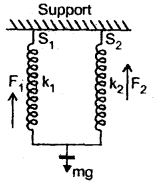The motion of the weight will be simple harmonic in nature. Its time period is given by
T = 2π$$\sqrt{\frac{m}{k}}$$
= 2π$$\sqrt{\frac{\mathrm{m}}{\mathrm{k}_{1}+\mathrm{k}_{2}}}$$

(b) When the springs are connected in series, the springs suffer different displacement y1 and y2 when the weight mg is pulled down. But the restoring force is the same in each spring.
∴ F = – k1y1
and F = -k2y2If k be the spring constant of the combination, then
F = -ky …..(4)
∴ From (3) and (4), we get
k = $$\frac{\mathbf{k}_{1} \mathbf{k}_{2}}{\mathbf{k}_{1}+\mathbf{k}_{2}}$$ …(5)

If ‘a’ be the acceleration produced in the body of mass m, then
a = $$\frac{\mathrm{F}}{\mathrm{m}}=\frac{-\mathrm{k}}{\mathrm{m}}$$y ⇒ motion of S.H.M. having time period T given by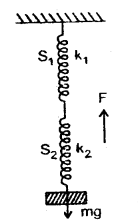(c) The weight ‘mg’ is connected in between the two springs which themselves are connected in series.

When the weight is pulled to one side, one spring gets compressed and the other is extended by the same amount. The restoring force acts in the same direction due to both the springs.

Let y = extension or compression produced in the springs,
F1, F2 = restoring forces produced in the springs, then
F1 = – k1y
F2 = – k2y
If F = F1 + F2 = – (k1 + k2)yIf k = spring constant of the combination, then
F = – ky;
∴ k = k1 + k2
a = $$\frac{F}{m}$$ = – $$\frac{\left(\mathrm{k}_{1}+\mathrm{k}_{2}\right)}{\mathrm{m}}$$y
and T = 2π$$\sqrt{\frac{M}{k}}$$ = 2π$$\sqrt{\frac{M}{k_{1}+k_{2}}}$$

Question 4.
(a) Obtain the expression for a time period of a simple pendulum.
(b) Calculate the total energy of a particle executing S.H.M.
(a) Let l = length of the string of the pendulum.
mg = weight of the bob acting vertically downward.
θ = angle by which the bob be displaced from the vertical position.
O = equilibrium position of the bob.

At any position P, the various forces acting on the bobs are:

1. mg, the weight of the bob acting in a vertically downward direction.
2. Tension (T) in the string acting along the string towards the support from which the pendulum is suspended.

mg is resolved into two rectangular components.

1. mg cos θ opposite to T.
2. mg sin θ perpendicular to T and directed towards the mean position O.T – mg cos θ provides the centripetal force = $$\frac{\mathrm{mv}^{2}}{l}$$ to the bob to move it in the circular path of radius l.

∴ T – mg cos θ = $$\frac{\mathrm{mv}^{2}}{l}$$
At extreme position P, bob is momentarily at rest i.e. v = 0

∴ T – mg cos θ = 0
or
T = mg cos θ ….(1)
mg sin θ provides restoring force (F), i.e.
F = – mg sin θ …. (2)

It acts towards the mean position. Here -ve sign shows that F tends to decrease θ. If θ is small, then
sin θ ~ θ
∴ F = – mg θ
Or
$$\frac{F}{m}$$ = – gθ
or
a = – gθ = – g. $$\frac{x}{l}$$ (∵ x = lθ)

where a = acceleration produced in the bob.
Now as a ∝ x and acts towards mean position, so its motion is S.H.M. having timC period (T) given by

T = 2π$$\sqrt{\frac{x}{a}}$$ = 2π$$\sqrt{\frac{l}{g}}$$

(b) A particle executing S.H.M. has two types of energy

1. P.E. due to its displacement from mean position.
2. K.E. due to its velocity.

Let M = mass of the particle executing S.H.M.
r = amplitude of S.H.M.
ω = angular frequency of S.H.M.
y = displacement after a time t. k = force constant of S.H.M.
F = ky, restoring force acting at any position A.

Let the body is displaced from A to B s.t. AB = dy
If dW = work is done on the body when displaced by dy, then
dW = F dy = ky dy

If W = work done when the body is displaced through y, then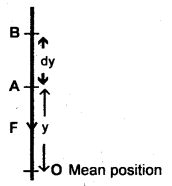This work is stored in the particle in the form of P.E.
i.e. E1 = P.E. = $$\frac{1}{2}$$ky2

KE. Let y = velocity of the particle executing S.H.M. at a displacement y from the mean position. Then
v = ω$$\sqrt{r^{2}-y^{2}}$$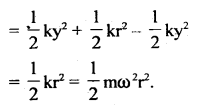Thus the total energy in S.H.M. remains constant.

Numerical Problems:

Question 1.
A particle executing S.H.M. along a straight line has a velocity of 4 ms-1 at a distance of 3 m from its mean position and 3 ms-1 at a distance of 4 m from it. Determine its angular speed and time period of oscillation.
Here, y1 = 3 m
v1 = 4 ms-1
y2 = 4 m
v2 = 3 ms-1
ω =?

We know that the velocity of a particle executing S.H.M. is given by∴ From (ii),
4 = ω $$\sqrt{25-9}$$ = 4ω
∴ ω = 1 rad s-1
∴ T = $$\frac{2π}{ω}$$ = 2π second.

Question 2.
A harmonic oscillation is represented by y = 0.34 cos (3000 t + 0.74) where y and t are in cm and s respectively. Deduce
(i) amplitude,
(ii) frequency and angular frequency,
(iii) time period,
(iv) initial phase.
The given equation is y 0.34 cos (3000 t + 0.74) …. (1)
Comparing eqn. (i) with the standard equation of displacement of harmonic oscillation,
y = r cos (ωt + Φo), we get
r = amplitude = 0.34 cmQuestion 3.
A particle vibrates simply harmonically with an amplitude of 4 cm.
(a) Locate the position of the point where its speed is half its maximum speed.
(b) At what displacement ¡s P.E. = K.E.?
Here, r = 4 cm
vmax = maximum speed of the particle
m = mass of the particle
(a) let v = speed of the particle when its displacement is y and
v = $$\frac{1}{2}$$vmax …(i)
Let y = required position w.r.t. mean pcsition =?

∴ maximum P.E = $$\frac{1}{2}$$kr2
Also maximum K.E = $$\frac{1}{2}$$mv2max

According to the law of conservation of energy,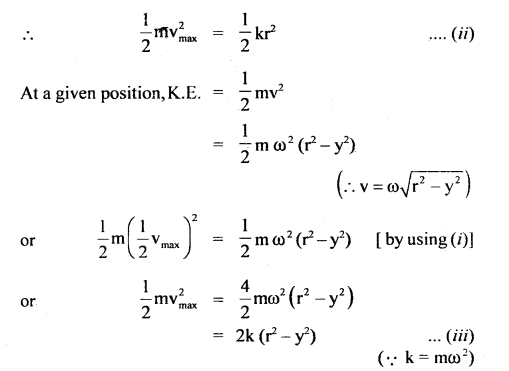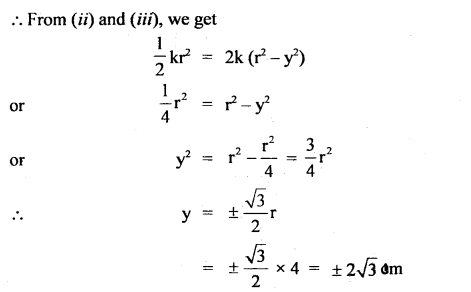(b) Let y1 be the displacement from the mean position at which
P.E.=KE.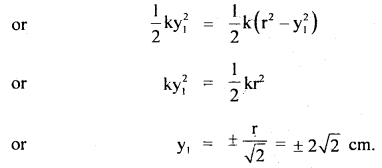Question 4.
A body of mass 12 kg is suspended by a coil spring of natural length 50 cm and force constant 2.0 × 103 Nm-1. What is the stretched length of the spring? 1f the body is pulled down further stretching the spring to a length of 59 cm and then released, what is the frequency of oscillation of the suspended mass? (Neglect the mass of the spring) .
Here, k = 2 × 103 Nm-1
m = 12 kg
L = original length of the spring
= 50 cm = 0.50 m

Let l = elongation produced in the spring when 12 kg mass is suspended.
∴ F = kl = mg
or
l = $$\frac{\mathrm{mg}}{\mathrm{k}}=\frac{12 \times 9.8}{2 \times 10^{3}}$$
= 0.0588 m = 5.88 cm

∴ stretched length of the spring = L + l = 50.00 + 5.88 = 55.88 cm

The time period of a loaded spring does not change even if the spring is further stretched. So when it is stretched to 59 cm, its time period will be given by
T= 2π$$\sqrt{\frac{m}{k}}$$

∴ Frequency of oscillation is given byQuestion 5.
A body of mass of 1.0 kg is suspended from a weightless spring having a force constant of 600 Nm-1. Another body of mass 0.5 kg moving vertically upward hits the suspended body with a velocity of 3.0 ms-1 and gets embedded in it. Find the frequency of oscillations and amplitude of motion.
Here, m1 = 1 kg
m2 = 0.5 kg
k = force constant = 600 Nm-1
v1 = 0
v2 = 3 ms-1

As the mass m2 gets embedded after collision; so
m = total mass = inertia factor = m1 + m2 = 1 + 0.5 = 1.5 kg
If v = frequency of oscillation, thenAccording to the law of conservation of linear momentum, pf = pi.
(m1 + m2)V = m1v1 + m2v2 = m2v2 (∵ v1 = 0)
∴ V = $$\frac{\mathrm{m}_{2} \mathrm{v}_{2}}{\mathrm{~m}_{1}+\mathrm{m}_{2}}=\frac{0.5 \times 3}{1.5}$$ = 1 ms-1

Let r = amplitude of motion
Also according to the law of conservation of mechanical energyQuestion 6.
(a) If the length of a second’s pendulum is increased by 1%, how many beats will it lose in one day?
(b) A pendulum clock normally shows the correct time. On an extremely cold day, its length decreases by 0.2%. Compute the error in time per day.
(a) Time period of a pendulum is
T = 2π$$\sqrt{\frac{l}{g}}$$

For second’s pendulum, T = 2s
∴ 2 = 2π$$\sqrt{\frac{l}{g}}$$ …(i)

Let l’ = new length of the second’s pendulum. When l is increased by 1%, then
l’ = l + l% of l = l +$$\frac{1}{100}$$l

If T’ = new time period, then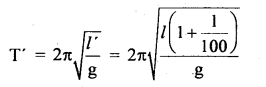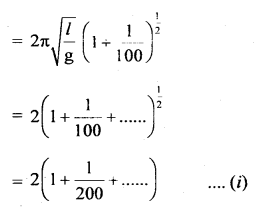(Using Binomial Expansion Theorem)
= 2 + $$\frac{1}{100}$$

No. of oscillations initially produced by the pendulum is
n = no. of seconds in one day/time
= $$\frac{24 \times 60 \times 60}{2}=\frac{86400}{2}$$ …(ii)

No. of oscillations produced by the pendulum after increasing its length.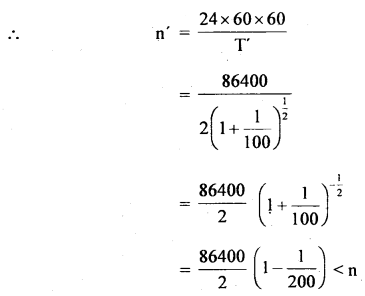Number of oscillations lost per day = no. of seconds lose per day
= 216 × 2 = 432 s.

(b) The correct time period of the pendulum clock 2s.
Let l be its correct length
∴ 2 = 2π$$\sqrt{\frac{l}{g}}$$ ….(iii)

Decrease in length = 0.2% of l = $$\frac{2}{100}$$l
If l’ be the new length than
l’ = l – $$\frac{0.2}{100}$$l = l(1 – $$\frac{0.2}{100}$$)

If T’ be the new time period, then= (2 – $$\frac{0.2}{100}$$) < 2
∴ Clock gains time,
Time gained in 2 seconds = $$\frac{0.2}{100}$$s

∴ Time gained per day = $$\frac{0.2}{100}$$ × $$\frac{1}{2}$$ × 24 × 60 × 60 = 86.4 s.

Question 7.
A simple pendulum is made by attaching a 1 kg bob to a 5 cm copper wire of diameter 0.08 cm and it has a certain period of oscillation. Next, a 10 kg bob is substituted for a 1 kg bob. Calculate the change in period if any. Young’s modulus of Copper is 12.4 × 1010 Nm-2.
Here, F = mg
A = πr²
The original length of the pendulum, l = 5 m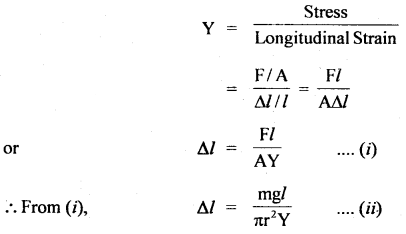Case I: m = 1 kg, l = 5 m, r = $$\frac{0.08}{2}$$ cm = 0.04 cm = 4 × 10-4 m, Y = 12.4 × 1010 Nm-2
∴ Δl1 = $$\frac{1 \times 9.8 \times 5}{3.14 \times(0.04)^{2} \times 12.4 \times 10^{10}}$$
= 0.0787 × 10-2 m
= 0.00079 m.

∴ Total length of the pendulum,
l1 = l + Δl1 = 5 m + 0.00079 m
= 5.00079 m.

If T1 be the initial time period of the pendulum, then
T1 = 2π$$\sqrt{\frac{l_{1}}{g}}$$
= 2 × $$\frac{22}{7} \sqrt{\frac{5.00079}{9.8}}$$
= 6.28 × 0.714 = 4.486 s

CaseII: M = 10kg, l = 5 m, r = 0.04 × 10-4m, Y = 12.4 × 1010 Nm-2
∴ Δl2 = $$\frac{10 \times 5 \times 9.8}{3.14 \times 16 \times 10^{-8} \times 12.4 \times 10^{10}}$$
= 0.0079 m
∴ l2 = l + Δl2 = 5 + 0.0079 = 5.0079 m

If T2 be the new time period of the pendulum, then
T2 = 2π$$\sqrt{\frac{l_{2}}{g}}$$
= 2 × 3.14$$\sqrt{\frac{5.00079}{9.8}}$$
= 4.489 s.

∴ Increase in time = T2 – T1 = 4.489 – 4.486 = 0.003s.

Question 8.
A second’s pendulum is taken in a carriage. Find the period of oscillation when the carriage moves with an acceleration of 4 ms-2.
(i) Vertically upwards,
(ii) Vertically downwards,
(iii) in a horizontal direction.
Here, T = 2s
using T = 2π$$\sqrt{\frac{l}{g}}$$, we get
∴ 2 = 2π$$\sqrt{\frac{l}{g}}$$
or
1 = 2π$$\frac{l}{g}$$
or
l = $$\frac{\mathrm{g}}{\pi^{2}}=\frac{9.8}{\pi^{2}}$$ …(i)

(i) When carriage moves up, a = 4 ms-2
∴ If T1 be the time period, then(ii) When the carriage moves down with a = 4 ms-2
If T2 be the time period, then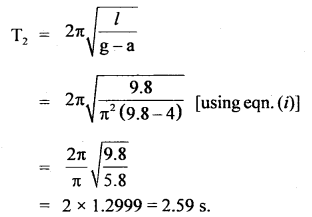(iii) When the carriage moves horizontally, then g and a are at right angles to each other and hence the net acceleration is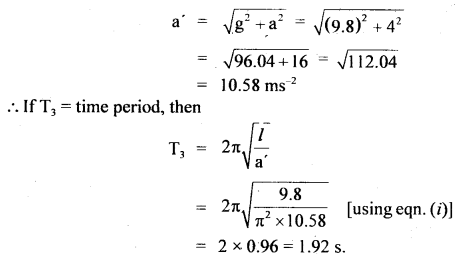Question 9.
A block is kept on a horizontal table. The table is undergoing S.H.M. of frequency 3 Hz in a horizontal plane. The coefficient of static friction between the block and the table surface is 0.72. Find the maximum amplitude of the table at which the block does not slip on the surface (g = 10 ms-2).
Here, v = 3 Hz.
μ = 0.72
g = 10 ms-2

Maximum acceleration of the block is
amax = rω2
where r = amplitude of the table
ω = its angular frequency = 2πv
= 2π × 3

∴ Maximum force on the block is given by
F = mamax = mrω2

Frictional force on the block,
fs = μmg

The block will not slip on the surface of the table if
F = fs
or
mrω2 = μmg
or
r = $$\frac{\mu g}{\omega^{2}}$$
or
r = $$\frac{0.72 \times 10.0}{(6 \pi)^{2}}$$ = 0.02 m

Question 10.
The pendulum bob has a speed of 3 ms-1 at its lowest position. The pendulum is 0.5 m long. What will be the speed of the bob when the length makes an angle of 60° with the vertical?
Let m = mass of the bob
y = speed of the bob at the lowest position = 3ms-1.l = length of the pendulum
= 0.5 m = $$\frac{1}{2}$$m

∴ K.E at the lowest position O = $$\frac{1}{2}$$mv2
= $$\frac{1}{2}$$m × 32 = $$\frac{9}{2}$$m …(i)

When the length (i) makes an angle O ( 60°) to the vertical, the bob of the pendulum will have both K.E. and P.E.

If v1 be the velocity of the bob in this position and h= height of the bob with respect to O, then
SC = l cos θ
∴ h = OS – SC = l – l cos θ
= l(l – cos θ)
= l(1 – $$\frac{1}{2}$$)
= $$\frac{l}{2}=\frac{1}{2} \times \frac{1}{2}$$m
= $$\frac{1}{4}$$m.

Total energy of the bob = K.E. + P.E.
= $$\frac{1}{2}$$mv12 + mgh
= $$\frac{1}{2}$$mv12 + m × 9.8 × $$\frac{1}{4}$$ …(iii)

∴ According to the law of conservation of energy,
K.E. at O = Total energy at A
$$\frac{9}{2}$$ m = $$\frac{1}{2}$$mv12 + $$\frac{\mathrm{m} \times 9.8}{4}$$
or
v12 = 9 – $$\frac{9.8}{2}$$ = 9 – 4.9 = 4.1
∴ v1 = 2.025 = 2.03 ms-1.

Question 11.
A person stands on a weighing machine placed on a horizontal platform. The machine reads 60 kg. When the platform executes S.H.M. at a frequency of 2.0 s-1 and amplitude 5.0 cm, what will be the effect on the reading of the weighing machine? Take g = 10 ms-2.
Here, m = 60 kg
v = 2 s-1
r = 5 cm = 0.05 m
ω = 2πv = 2π × 2 = 4π rad-1 s

Here A and B are the extreme positions between which the platform vibrates and O = mean position.
∴ acceleration is maximum at A or B and is given by
amax = rω2 = 0.05 × (4π)2
= 0.05 × 16 × 9.87
= 7.9 ms-2

∴ restoring force, F = mamax
= m × 7.9 N
= 7.9 m N

At point A, both weight and restoring force are directed towards the mean position, so the effective weight is maximum at A i.e. reading will be maximum and is given by
W1 = mg + mamax = m(g + amax)
= 60 (10 + 7.9)
= 60 × 17.9 N
= 1074.0 N
= 107.4 kgf

At point B, the weight and the restoring force are opposite to each other, so the effective weight i.e. reading of the weighing machine is minimum at B and is given by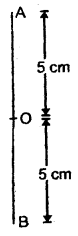Question 12.
If earth were a homogeneous sphere and a square hole was bored in it through its center, show that a body dropped in the hole will execute S.H.M. and calculate its period if the radius of earth = 6400 km.
Let R = radius of earth 6400 × 103 m
O = center of the earth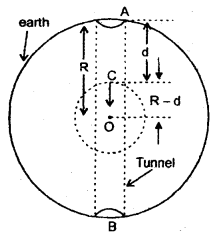Let AB be a tunnel bored along the diameter of the earth and a body be dropped from point A.

Let it reaches point C at a depth d from the surface of the earth.
∴ OC = R — d = distance of the object from the center of earth displacement of the body.

If gd be the acceleration due to gravity at a depth ‘d’ then we know, that
gd = g(1 – $$\frac{d}{R}$$) = g\frac{(R-d)}{R}

If R – d = y = displacement from O, then
gd = $$\frac{g}{R}$$y

Now as the acceleration of the body is proportional to its displacement from the center of earth i.e. O. Hence the motion of the body is S.H.M. and its time period is given byQuestion 13.
A horizontal spring block system of mass M executes S.H.M. When the block is passing through its equilibrium position, an object of mass m is put on it and the two move together. Find the new amplitude and frequency of vibration.
The frequency of oscillation of spring block system of mass M is given by
v = $$\frac{1}{2 \pi} \sqrt{\frac{\mathrm{k}}{\mathrm{M}}}$$ …..(i)
where k = force constant of the spring.

Let r = initial amplitude of oscillation.
Vo = velocity of the system in equilibrium position,
according to the law of conservation of energy,
∴ $$\frac{1}{2}$$MV02 = $$\frac{1}{2}$$kr2
or
Vo = $$\sqrt{\frac{k}{M}}$$r ….(ii)

When mass m is put on the system, then total mass = M + m.

If V = velocity of the combination in equilibrium, then according to the law of conservation of linear momentum,
MVo = (M + m)V
or
V = $$\frac{\mathrm{MV}_{0}}{\mathrm{M}+\mathrm{m}}$$ ….(iii)

Let r1 be the new amplitude, then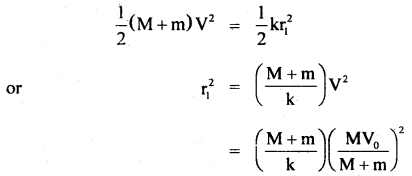If v’ be the new frequency of oscillation, then
v’ = $$\frac{1}{2 \pi} \sqrt{\frac{\mathrm{k}}{\mathrm{M}+\mathrm{m}}}$$

Question 14.
A tray of mass 12 kg is supported by two parallel vertical identical springs. When the tray is pressed down slightly and released, it executes S.H.M. with a time period of 1.5 s. What is the force constant of each spring? When a block of mass m is placed in the tray, the period of S.H.M. changes to 3 s. What is the mass of the block?
Let k be the spring constant of each of the identical springs connected in parallel.
M = mass of tray = 12 kg
T1 = time period of oscillation of the tray = 1.5 s

Let T2 = time period of oscillation of the tray + block of mass ‘m’
∴ T2 = 3 s
m = ?
k =?

If k’ = spring constant of the combination, then
k’ = k + k = 2k (a)Dividing (ii) and (i), we get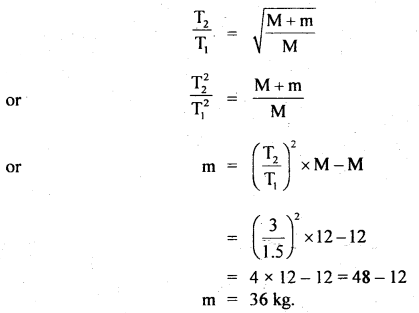Question 15.
Two pendulums of lengths 100 cm and 110.25 cm start oscillating in phase simultaneously. After how many oscillations will they again be in phase together?
Here, l1 = 100 cm
l2 = 110.25 cm
using T = 2π$$\sqrt{\frac{l}{g}}$$

For smaller pendulum, T1 = 2π$$\sqrt{\frac{100}{g}}$$ …(i)

For larger pendulum, T2 = 2π$$\sqrt{\frac{110.25}{g}}$$ …(ii)
where T1 and T2 are the time periods of the two pendulums.

Let these pendulums oscillate in phase again if the larger pendulum completes n oscilLations, It means the smaller pendulum must complete (n + 1) oscillations.
nT2 = (n + 1)T1Hence both pendulums will again oscillate in phase after 20 oscillations of the larger or 21 oscillations of the smaller pendulum.

Question 16.
Springs of spring constants k, 2k, 4k., 8k, are connected in series. A mass m kg ¡s attached to the lowér end to the last spring and the system is allowed to vibrate. Calculate the approximate time period of vibration.
The time period of vibration of series loaded spring is given by
T = 2π$$\sqrt{\frac{\mathrm{m}}{\mathrm{k}_{\mathrm{s}}}}$$ …(1)
Where k is the force constant of the series combination of springs.

In a series combination of springs, the combined spring constant k is given by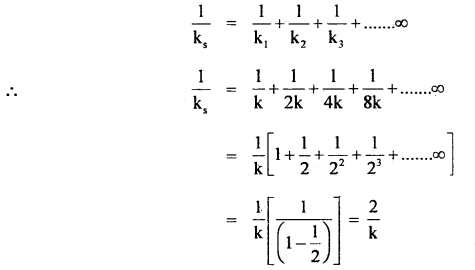or
ks = $$\frac{k}{2}$$

m mass attached to the lower end of the spring.
∴ From (1) and (2), we get
T = 2π$$\sqrt{\frac{2 m}{k}}$$

Question 17.
A point particle of mass 0.1 kg is executing S.H.M. of amplitude 0.1 m. When the particle passes through the mean position, its kinetic energy is 8 × 10-2 J. Obtain the equation of motion of this article if the initial phase of oscillation is 45°
Since the kinetic energy is maximum at the mean position and is equal toThe standard equation of motion of a particle executing S.H.M. is
y = A sin(ωt + Φ2)
or
x = A cos(ωt + Φ2)
Given r = 0.1 m, ω = 4 rad s-1 and Φ2 = 45° = $$\frac{π}{4}$$

∴ The required cquation of motion is
y = 0.1 sin (4t + $$\frac{π}{4}$$)
or
x = 0.1 cos (4t + $$\frac{π}{4}$$).

Question 18.
A cubical body (side 0.1 m and mass 0.002 kg) floats in water. It is pressed and then released so that it oscillates vertically. Show that the motion of the body is simple harmonic and And the time period.
Here, m = mass of body = 0.002 kg
L = side of body = 0.1 m
In the absence of any given indication it is assumed that the body floats (just sunk), so

The mass of water displaced = volume × density of water
= V ρ = (0.1 )3 ρ.

The upward thrust exerted by water displaced
i.e. buoyant force = Vρg=(0.1 )3 × 103 × 9.8 (∴ ρ ofwater = 103 kg m3)
W1 = Downward weight = mg
Net downward force, F = (0.002 g -Vρg)
or
F = [0.002g – (1)3 × 103g
= (0.002 – 1)g
= – 0.998 × 9.8 N …. (1)

Suppose the block is depressed by y,
hence restoring force F1 = – (0.1 + y)k

When the body floats on the water the force acting is F = 0.1 k, where k is the force constant
The net downward force
F – F’ = 0.1 k – (0.1 + y)k
= – yk ….(2)

Thus force ∝ (- y)
Force = ma, where a = acceleration of the body.
∴ ma = – y k
or a = – $$\frac{k}{m}$$ y
m
or a ∝ -y …. (3)

Hence the motion-ofthe block is S.H.M.
The time period of oscillation is given by
T = 2π$$\frac{m}{k}$$ …(4)

From eqn. (1), k = $$\frac{\mathrm{F}}{\mathrm{L}}=\frac{0.998 \times 9.8}{0.1}$$Nm-1 …(5)
m = 0.002 kg

∴ T = 2π$$\sqrt{\frac{0.002 \mathrm{~kg} \times 0.1}{0.998 \times 9.8 \mathrm{Nm}_{1}}}$$
= 0.0284 s.

Question 19.
Two simple harmonic motions are represented by the following equations:
y1 = 10 sin $$\frac{π}{4}$$ (12t + 1); y2 = 5 (sin 3πt + $$\sqrt{3}$$ cos 3πt).
(a) Find out the ratio of their amplitudes.
(b) What are the time periods of two motions?
(c) Also find the phase difference between two motions.
The two given displacements may be written as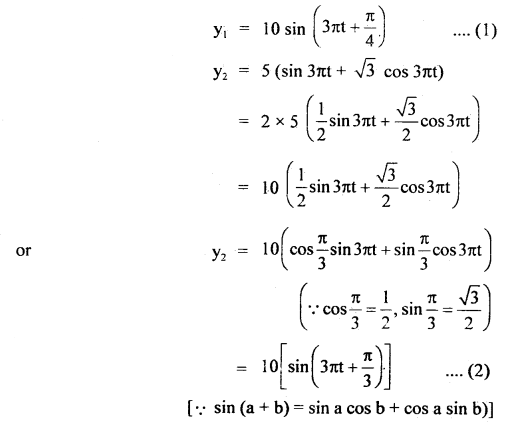(a) The amplitudes of the two S.H.M. are A1 = 10 units. and A2 = 10 units
Hence $$\frac{\mathrm{A}_{1}}{\mathrm{~A}_{2}}=\frac{10}{10}$$ = 1

(b) Comparing the given equation with the normal sine function of S.H.M.
i.e. y = A sin($$\frac{2πt}{T}$$ + Φ) …(3)

Here we get $$\frac{2πt}{T}$$ = 3πt
or
T = $$\frac{2}{3}$$ s

i.e. Time period for two motions is same i.e. T = 0.67 s.

(c) Phase difference between two motions:
Phase (Φ1) of motion represented by y1 is
Φ1 = 3πt + $$\frac{π}{4}$$ …(4)

Phase (Φ2) of motion represented by y2 is
Φ2 = 3πt + $$\frac{π}{3}$$ …(5)

∴ Phase difference is given by
dΦ = Φ2 – Φ1 = (3πt + $$\frac{π}{3}$$) – (3πt + $$\frac{π}{4}$$)
= (4 – 3)$$\frac{π}{12}$$
= $$\frac{π}{12}$$ = 15°

Question 20.
Two particles execute- S.H.M. of the same amplitude at time period along the same straight line. They cross each other in opposite directions at half the amplitude. What is the phase difference between them?
Let the displacement of one particle executing S.H.M. be
y1 = A sin ωt …. (1)

when y1 = $$\frac{A}{2}$$
From equation (1), we get.
$$\frac{A}{2}$$ = A sin ωt
or
sin ωt = $$\frac{1}{2}$$ = sin 30°
∴ ωt = 30° = $$\frac{π}{6}$$ radian.

If Φ1 be the phase of first particle then Φ = $$\frac{π}{6}$$ radian. Also let the displacement of second particle executing S.H.M. be
y1 = r sin (π – ωt) = r sin Φ2 ….(2)
where Φ2 = π – ωt = π – $$\frac{π}{6}$$ = $$\frac{5}{6}$$π radian

If dΦ be the phase difference between the two particle when they cross each other, then
dΦ = Φ2 – Φ1
= $$\frac{5}{6}$$π – $$\frac{π}{6}$$ = $$\frac{4π}{6}$$ = $$\frac{2}{3}$$π radian
= 120°.

Question 21.
A spring compressed by 10 cm develops a restoring force of 5N. A body of mass of 2 kg is placed on it. Find
(i) Force constant,
(ii) depression of a spring,
(iii) time period and
(iv) the frequency of oscillations, if the body is disturbed.
Here, F = 5N
x = 10 cm = 0.1 m
m = 2 kg
(i) k = force constant = $$\frac{F}{x}=\frac{5 N}{0.1}$$ = 50 Nm-1.

(ii) depression, l = $$\frac{\mathrm{mg}}{\mathrm{k}}=\frac{2 \times 9.8}{50}$$ = 0.392 m

(iii) T = 2π$$\sqrt{\frac{m}{k}}$$ = 2π$$\sqrt{\frac{2}{50}}$$ = $$\sqrt{\frac{2π}{5}}$$s

(iv) v = $$\frac{1}{\mathrm{~T}}=\frac{5}{2 \pi}$$ Hz

Question 22.
The S.H.M. of a particle is given by the equation y = 3 sin cot + 4 cos cot. Find its amplitude.
The equation y = 3 sin ωt + 4 cos ωt is due to the superposition of two waves,
y1 = 3 sin ωt
and
y2 = 4 cos ωt = 4 sin(ωt + $$\frac{π}{2}$$)

If r1 and r2 be the amplitudes of the two w’aves respectively, then r1= 3 units and r2 = 4 units.
Φ = phase difference between them = $$\frac{π}{2}$$

If r be the amplitude of the resultant wave, then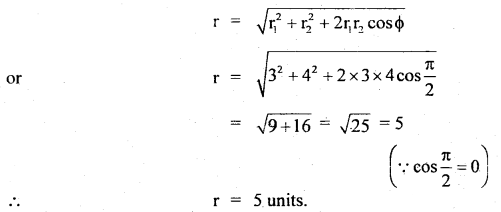Question 23.
Two masses m1 = 1.0 kg and m2 = 0.5 kg arc suspended together by a massless spring of spring constant k as shown in the figure. When masses are in equilibrium, m, is removed without disturbing the system. Calculate the amplitude of oscillation and angular frequency of m2. (g = 10 ms-2 and k = 12.5 Nm-1).
Let y = extension in length of the spring when both m1 and m2 are suspended with it, then
(m1 + m2)g = ky
or
y = $$\frac{\left(m_{1}+m_{2}\right) g}{k}$$ …(1)

Let y’ extension in the length of the spring when only m is suspended.
∴ m2g = ky’
or
y’ = $$\frac{\mathrm{m}_{2} \mathrm{~g}}{\mathrm{k}}$$ …(2)

(1) – (2) gives y – y’ = $$\frac{\left(m_{1}+m_{2}\right) g}{k}-\frac{m_{2} g}{k}=\frac{m_{1} g}{k}$$

This represents the amplitude of oscillation (r)
i.e. r = y – y’ = $$\frac{\mathrm{m}_{1} \mathrm{~g}}{\mathrm{k}}$$ …(3)
Here, m1 = 1.0 kg
k = 12.5 Nm-1
g = 10 ms-2

Putting these values in equation (3), we get
r = $$\frac{1 \times 10}{12.5}$$ = 0.8 mIf ω be the angular frequency of m2, then
ω = $$\sqrt{\frac{\mathrm{k}}{\mathrm{m}_{2}}}=\sqrt{\frac{12.5}{0.5}}$$ (∵ m2 = 0.5 kg)
ω = 5 s-1.

Question 24.
Prove that T = 84.6 minutes for a simple pendulum of infinite length.
T = 2π$$\sqrt{\frac{l}{g}}$$ …(1)
eqn. (1) hold good till l << R (radius of the earth).

When l is large, the curvature of the earth had also to be taken into account. In that case,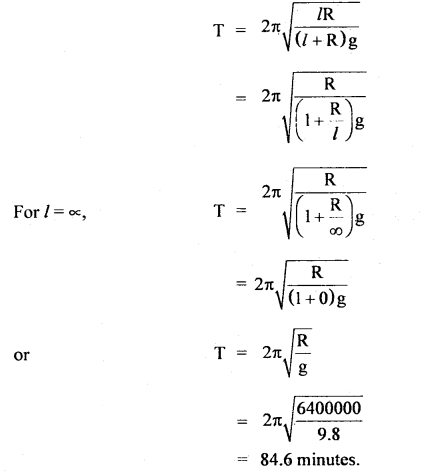Question 25.
For damped oscillator, m = 200 gm, k = 90 Nm b = 40 gs-1.
Calculate (a) time period of oscillation,
(b) time is taken for its amplitude of vibration to become $$\frac{l}{2}$$ of its initial value.
(c) time is taken for its mechanical energy to drop to half of its initial value.
Here, m = 200 g = 0.200 kg
k = 90 Nm-1
b = 40gs-1.
(a) $$\sqrt{km}$$ = $$\sqrt{90 \times 0.2}=\sqrt{18}$$ = 4.243 kg s-1
b = 0.04 kg s-1
∴ b << $$\sqrt{km}$$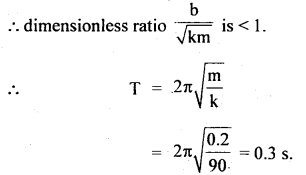(b) Let T1/2 = time taken for the amplitude to drop to half of its initial value.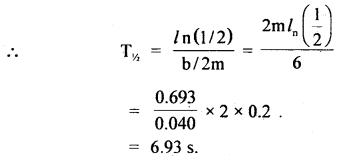(c) Let T’1/2 be the time for its mechanical energy to drop to half of its initial value,
∴ Using equation,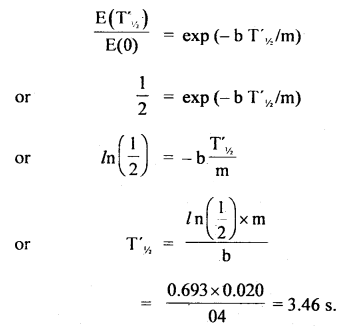which is just half of T1/2. (i.e. decay period for amplitude).

Value-Based Type:

Question 1.
A sports teacher was training the children to march- past On their way they come across a bridge. Then the physical education teacher stopped the children from marching on the bridge.
(a) Comment upon the values of sports teachers.
The sports teacher is responsible, cares not only for public property but also for children.

(b) Also explain what is meant by Resonance.
When the frequency of marching coincides with the natural frequency of oscillation of the bridge then the bridge oscillates with maximum amplitude to such an extent that the bridge may even collapse. The condition is called “Resonance”.

Question 2.
The Physics teacher of class XI has assigned the work finding the resultant spring constant when two springs of spring constants K1, K2 are joined in series. Two students Sabita and Shirin. Sabita made a theoretical study as well as verified it experimentally. Whereas Shirin could not complete the work. When th~ teacher enquired the next day Sabita could give the answer. Whereas Shirin could not.
(a) Comment upon the qualities of Sabita.
Sabita is Sincere and hardworking and has a scientific temper.

(b) Two springs are joined in series and connected to a mass m. If spring constants are Kj and K2, Calculate the period of oscillation of mass m.
Since K1 and K2 are joined in series.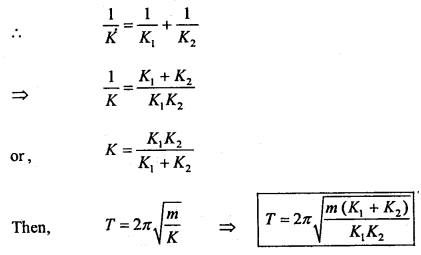Question 3.
Adarsh a student of class XI has found the factors on which the time period of oscillation of a pendulum depends and arrived at the expression T – (constant) × (l/g)1/2. He wants to know how the length of the pendulum gets affected on the surface of the moon for the same pendulum and arrived at the conclusion that it is 1/6.
(a) What values does Adarsh possesses?
Adarsh is hardworking, thinks logically, having a scientific temper, able to find solutions with patience.

(b) The length of a second pendulum on the surface of the moon?
Since l is proportional to ‘g’ the pendulum on the surface of the moon will be 1/6m.

Question 4.
In a physics class, the teacher told that everyone has to finish the homework and assignment within a weak. But only five students including Dinesh did their homework on time. A few questions from the assignment are as under:
(i) Derive the expression for velocity in S.H.M.